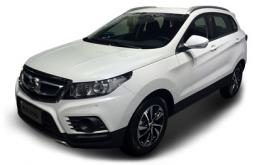# 北汽绅宝绅宝X55

## 当物超所值巧遇唾手可得 绅宝X55全包一口价

2017年09月14日 11:33来源：车行天下超过：9528次关注

人人都希望买到一件物超所值的商品，然而好东西并不那么容易就能到手。设想当“物超所值”变得唾手可得，那会是什么样子呢？绅宝X55就是如此，北汽绅宝的品质和定位令其物超所值，而“全包一口价”的购车政策则让它唾手可得。此时你会发现生活是美好的，日子是有盼头儿的，好东西终究是买得起的。常言道：“年轻人！好日子还在后头呢！”物超所值X55

说到这里可能有人会问了，绅宝X55是何许车也？何德何能配得上物超所值的头衔？现如今国内SUV市场的竞争愈演愈烈，优秀的产品层出不穷，X55想要瓜分市场必须有几把刷子。

我相信首先从外观层面它就能抓住消费者的目光，绅宝家族标志性的前进气格栅可识别度很高，侧身错落的腰线进一步提升车辆的动态感。环绕车身四周的黑色下裙，再点缀车身颜色的同时，也能保护车身在走拦路时不被障碍物擦伤。车尾下方双边单出的排气管再加上银色金属材质护板，则令其看上去多了一些越野气质。作为一款定位紧凑级SUV的产品，X55凭借4405*1809*1685毫米的三围和2650毫米的轴距为驾乘者提供了充分的内部空间。还有一点我忘说了，尽管X55是城市SUV，但200毫米的离地间隙也足够拿出来炫耀，因为这比奥迪Q5（185毫米）的还要高。X55能有赶超合资豪华品牌产品的硬件设定，还是很有面子的。200毫米的离地间隙确保了车辆的通过性

作为国产自主品牌车型，没有琳琅满目的配置和令人心旷神怡的全景天窗，都不好意思跟人打招呼！像车身稳定系统、上坡辅助、胎压监测什么的早就不在话下了，自动大灯、手机互联、自动雨刷、无钥匙进入/启动、倒车影像、智能车载语音系统等配置基本上也已经追平了合资品牌车型。自然吸气和涡轮增压版车型分别搭载了116马力和150马力的发动机，它们的排量都是1.5升。除此之外还有CVT无级变速、6挡和5挡手动变速器可选。可见在硬件方面留给消费者的选择余地还是很大的。此时估计有有人会问，你这尺寸赶超合资品牌、配置怒怼同级对手、动力满足众人所求的绅宝X55看上去是要上天的节奏哇，这得多少钱呢？答曰：价格区间涵盖7.68-11.98万元。买得起不？这两年好像大家的收入都在上涨，十万左右的支出似乎压力不大。所以说X55已经算得上物超所值了。

诚意“全包一口价”买车花的是大钱，全款提车需要时间攒钱，贷款是个解决燃眉之急的好办法，然而很多消费者等到缴纳税险、办理车牌的时候才发现手头儿银子就差那么一点儿又不够了。为了缓解客户这种尴尬，北汽绅宝推出了“全包一口价”的购车政策，将全额车款、保险、购置税相关费用整体打包，用户只需支付市场指导价10%的首付款就能将爱车“贷(带)”回家，完全不用担心预算不足的问题；此外北汽绅宝还为用户提供了远低于市场费率水平的贴息优惠，月供更是最低至1707元，将购车成本一省到底。好货不再难觅，新车唾手可得，还有什么理由不出手呢？眼看“金九银十”最佳购车季已经到来，当你在车市看花了眼、遛瘸了腿的时候，不妨关注一下各大厂商的购车优惠政策。相信不会再有比北汽绅宝X55再具诚意了。所以，年轻人！抓紧时机趁早开启美好的有车生活吧

#### 相关文章

0-500 字已有评论 0条 查看评论>>

### 绅宝X55### 猜你喜欢

﻿
• 快速找车
• 选择品牌
• 选择品牌
• A  奥迪
• A  阿斯顿·马丁
• A  阿尔法·罗密欧
• B  宝沃
• B  布加迪
• B  巴博斯
• B  保时捷
• B  宾利
• B  奔驰
• B  宝马
• B  本田
• B  别克
• B  标致
• B  比亚迪
• B  宝骏
• B  北汽制造
• B  北汽新能源
• B  北汽幻速
• B  北汽威旺
• B  北京汽车
• B  奔腾
• B  北汽绅宝
• C  长安
• C  长安商用
• C  长城
• C  昌河
• D  大众
• D  道奇
• D  DS
• D  东南
• D  东风风神
• D  东风风行
• D  东风小康
• D  东风风度
• D  东风
• F  福特
• F  丰田
• F  菲亚特
• F  法拉利
• F  福田
• F  福迪
• F  福汽启腾
• G  观致
• G  广汽传祺
• G  广汽吉奥
• G  GMC
• H  红旗
• H  汉腾汽车
• H  哈弗
• H  哈飞
• H  海格
• H  海马
• H  华颂
• H  黄海
• H  华泰
• H  恒天
• J  吉利汽车
• J  捷豹
• J  Jeep
• J  江淮
• J  江铃
• J  金杯
• J  九龙
• J  金旅
• K  凯翼
• K  凯迪拉克
• K  克莱斯勒
• K  科尼塞克
• K  卡威
• K  开瑞
• L  路虎
• L  林肯
• L  劳斯莱斯
• L  兰博基尼
• L  雷克萨斯
• L  铃木
• L  雷诺
• L  理念
• L  力帆
• L  莲花汽车
• L  猎豹
• L  路特斯
• L  陆风
• M  马自达
• M  MG
• M  MINI
• M  玛莎拉蒂
• M  摩根
• M  迈凯轮
• N  纳智捷
• O  欧宝
• O  讴歌
• O  欧朗
• Q  奇瑞
• Q  起亚
• Q  启辰
• R  日产
• R  荣威
• R  瑞麒
• S  三菱
• S  斯威汽车
• S  萨博
• S  smart
• S  斯柯达
• S  斯巴鲁
• S  思铭
• S  双龙
• S  上汽大通
• S  双环
• T  特斯拉
• T  腾势
• W  沃尔沃
• W  五菱汽车
• W  五十铃
• W  威兹曼
• W  威麟
• X  现代
• X  雪佛兰
• X  雪铁龙
• X  西雅特
• Y  一汽
• Y  英菲尼迪
• Y  英致
• Y  依维柯
• Y  野马汽车
• Y  永源
• Z  众泰
• Z  中华
• Z  中兴
• Z  知豆
• 选择车系
• 选择车系
• 车型对比
• 选择品牌
• 选择品牌
• A  奥迪
• A  阿斯顿·马丁
• A  阿尔法·罗密欧
• B  宝沃
• B  布加迪
• B  巴博斯
• B  保时捷
• B  宾利
• B  奔驰
• B  宝马
• B  本田
• B  别克
• B  标致
• B  比亚迪
• B  宝骏
• B  北汽制造
• B  北汽新能源
• B  北汽幻速
• B  北汽威旺
• B  北京汽车
• B  奔腾
• B  北汽绅宝
• C  长安
• C  长安商用
• C  长城
• C  昌河
• D  大众
• D  道奇
• D  DS
• D  东南
• D  东风风神
• D  东风风行
• D  东风小康
• D  东风风度
• D  东风
• F  福特
• F  丰田
• F  菲亚特
• F  法拉利
• F  福田
• F  福迪
• F  福汽启腾
• G  观致
• G  广汽传祺
• G  广汽吉奥
• G  GMC
• H  红旗
• H  汉腾汽车
• H  哈弗
• H  哈飞
• H  海格
• H  海马
• H  华颂
• H  黄海
• H  华泰
• H  恒天
• J  吉利汽车
• J  捷豹
• J  Jeep
• J  江淮
• J  江铃
• J  金杯
• J  九龙
• J  金旅
• K  凯翼
• K  凯迪拉克
• K  克莱斯勒
• K  科尼塞克
• K  卡威
• K  开瑞
• L  路虎
• L  林肯
• L  劳斯莱斯
• L  兰博基尼
• L  雷克萨斯
• L  铃木
• L  雷诺
• L  理念
• L  力帆
• L  莲花汽车
• L  猎豹
• L  路特斯
• L  陆风
• M  马自达
• M  MG
• M  MINI
• M  玛莎拉蒂
• M  摩根
• M  迈凯轮
• N  纳智捷
• O  欧宝
• O  讴歌
• O  欧朗
• Q  奇瑞
• Q  起亚
• Q  启辰
• R  日产
• R  荣威
• R  瑞麒
• S  三菱
• S  斯威汽车
• S  萨博
• S  smart
• S  斯柯达
• S  斯巴鲁
• S  思铭
• S  双龙
• S  上汽大通
• S  双环
• T  特斯拉
• T  腾势
• W  沃尔沃
• W  五菱汽车
• W  五十铃
• W  威兹曼
• W  威麟
• X  现代
• X  雪佛兰
• X  雪铁龙
• X  西雅特
• Y  一汽
• Y  英菲尼迪
• Y  英致
• Y  依维柯
• Y  野马汽车
• Y  永源
• Z  众泰
• Z  中华
• Z  中兴
• Z  知豆
• 选择车系
• 选择车系
• 选择车型
• 选择车型
• 意见反馈